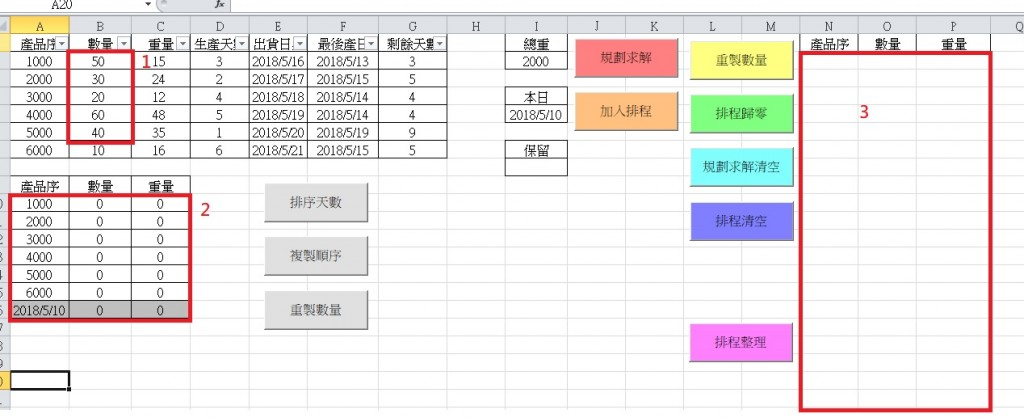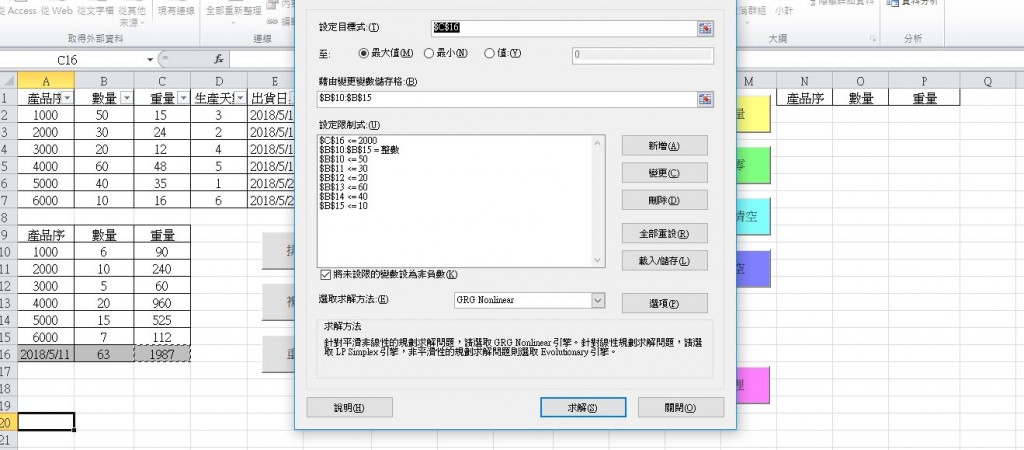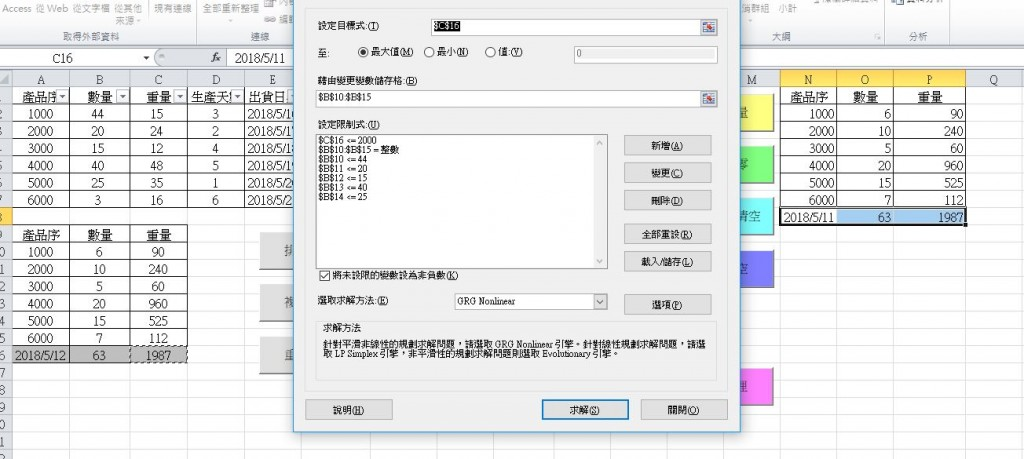#1

1. 限制條件
2. 規劃求解解出來的內容
3. 排程區``````Private Sub CommandButton1_Click()

Dim i As Integer

Dim DD As Date

Worksheets("工作表1").Activate
SolverReset

If Application.WorksheetFunction.Sum(Range("B2:B7")) <= 0 Then
MsgBox "沒有訂單了!!"
Exit Sub
End If

i = 2

Do Until i > 7

If Cells(i, 2) > 0 Then
SolverAdd cellRef:=Range("B" & i + 8), _
relation:=1, _
formulaText:=Cells(i, 2)
Else
SolverAdd cellRef:=Range("B" & i + 8), _
relation:=2, _
formulaText:=0
End If

i = i + 1

Loop

SolverOk SetCell:=Range("C16"), _
MaxMinVal:=1, _
ByChange:=Range("B10:B15")
Engine = 3

relation:=4

relation:=1, _
formulaText:=Cells(2, 9)

SolverSolve userFinish:=True
SolverFinish KeepFinal:=1

DD = Cells(16, 1)

Cells(16, 1) = DD + 1

End Sub
``````